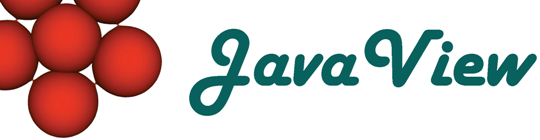Overview  Gallery  Introduction  Objects  Packages  Packages 3D  Basic Options  3D Options  Advanced

## 7. Working with Advanced Options

#### Prolog and Epilog

 Prolog and Epilog are options for graphics functions which gives a list of graphics primitives to be rendered before resp. after the main part of the graphics is rendered. In:= pe = Graphics3D[Stellate[Dodecahedron[]], Prolog -> {RGBColor[0., 0., 0.], Polygon[{{1., 1.}, {1., 0.}, {0., 1.}}]}, Epilog -> {RGBColor[1., 0., 0.], Thickness[0.02], Line[{{0.6, 0.9}, {0.776, 0.357}, {0.315, 0.693}, {0.885, 0.693}, {0.424, 0.357}, {0.6, 0.9}}]}]; Show[pe]In:= JavaView[pe] Compare with Mathematica

#### Background

 Specifies a backgrond color. In:= bg = Graphics3D[Stellate[Dodecahedron[]], Background -> RGBColor[1., 0., 0.]]; Show[bg]In:= JavaView[bg] Compare with Mathematica

#### DefaultColor

 DefaultColor is an option for graphics functions which specifies the default color to use for lines, points, etc. In:= dc = Graphics3D[Stellate[Dodecahedron[]], DefaultColor -> RGBColor[1, 0, 0]]; Show[dc]In:= JavaView[dc] Compare with Mathematica

#### PlotRegion

 PlotRegion is an option for graphics functions that specifies what region of the final display area a plot should fill. In:= pr = Graphics3D[Stellate[Dodecahedron[]], PlotRegion -> {{0., 0.5}, {0., 1.}}]; Show[pr]In:= JavaView[pr] Compare with Mathematica

#### TextStyle

 TextStyle is an option for graphics functions and for Text which specifies the default style and font options with which text should be rendered. In:= ts = Graphics3D[Stellate[Dodecahedron[]], TextStyle -> {FontSlant -> "Italic", FontSize -> 12}, Axes -> True]; Show[ts]In:= JavaView[ts] Compare with Mathematica

#### FormatType

 FormatType is an option for output streams,graphics and functions such asText which specifies the default format type to use when outputting expressions. In:= ft = Graphics3D[Stellate[Dodecahedron[]], FormatType -> TraditionalForm, Axes -> True, Ticks -> {{-1, -1/2, 0, 1/2, 1}, {-1, -1/2, 0, 1/2, 1}, {-1, -1/2, 0, 1/2, 1}}]; Show[ft]In:= JavaView[ft] Compare with Mathematica

#### ColorFunction and ColorFunctionScaling

 ColorFunction is an option for various graphics functions which specifies a function to apply to a values to determine the color to use for a particular a, a region. In:= cf = SurfaceGraphics[Table[Sin[x]*Exp[y], {x, -Pi, Pi, Pi/4.}, {y, -E, E, E/4.}], ColorFunction -> Automatic, Lighting -> False]; Show[cf]In:= JavaView[cf] Compare with Mathematica

#### RenderAll

Shows no effect in JavaView. RenderAll is an option for the Postscript export of Mathematica and has no visible effects in the Mathematica display.

#### ColorOutput

 ColorOutput is an option for graphics functions which specifies the type of color output to produce. In:= co = Graphics3D[Stellate[Dodecahedron[]], ColorOutput -> GrayLevel]; Show[co]In:= JavaView[co] Compare with Mathematica

#### ImageSize

The ImageSize is used as display size, when JavaView runs as application. If it is used in an applet, the applet tag defines the size of the display and the ImageSize option is ignored.

#### FaceForm

 FaceForm is a directive which specifies separate color directives to be used for drawing the front and back faces of a geometry. In:= ff = Graphics3D[{FaceForm[RGBColor[0., 0., 1.], RGBColor[1., 0., 0.]], Stellate[Dodecahedron[]]}, Lighting -> False]; Show[ff]In:= JavaView[ff] Compare with Mathematica

#### EdgeForm

 EdgeForm[ ] specifies the one or more graphics directives to be used for the drawing of edges. In:= ef = Graphics3D[{EdgeForm[RGBColor[1., 0., 0.], Thickness[.01]], Stellate[Dodecahedron[]]}]; Show[ef]In:= JavaView[ef] Compare with Mathematica